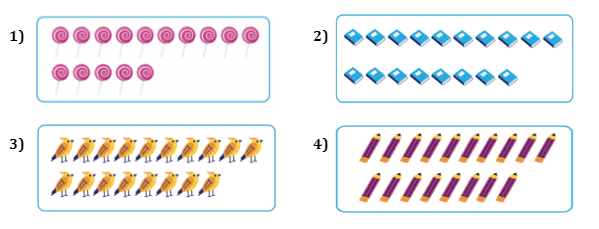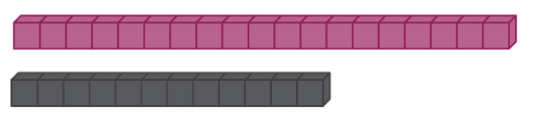Study P1 Mathematics Numbers to 20 - Geniebook# Numbers To 20

In this article, we will learn Numbers to 20 and focus on:

1. Counting to 20
2. Reading and Writing numbers in numerals and words
3. Comparing and ordering of numbers

## 1. Counting To 20• 10 and 1 make 11 or Eleven
• 10 and 2 make 12 or Twelve
• 10 and 3 make 13 or Thirteen
• 10 and 4 make 14 or Fourteen
• 10 and 5 make 15 or Fifteen•  10 and 6 make 16 or Sixteen
• 10 and 7 make 17 or Seventeen
• 10 and 8 make 18 or Eighteen
• 10 and 9 make 19 or Nineteen
• 10 and 10 make 20 or Twenty

## 2. Reading And Writing Numbers In Numerals And Words

Question 1:

Fill in the blanks with the correct numerals.

 Numerals Words 11 12 13 14 15 16 17 18 19 20

 Numerals Words 11 Eleven 12 Twelve 13 Thirteen 14 Fourteen 15 Fifteen 16 Sixteen 17 Seventeen 18 Eighteen 19 Nineteen 20 Twenty

Question 2:

There are __________ girls in the picture below.1. 11
2. 12
3. 13
4. 14

(2) 12

Solution:There are 10 girls in the box. Count on starting from 10:

11, 12.

Question 3:There are __________ ladybugs in the picture below.

1. 15
2. 18
3. 19
4. 20

(1) 15

Solution:There are 10 ladybugs in the box.  Count on from 10:

11, 12, 13, 14, 15.

Question 4:

Count and write the number of stars in words.1. Eleven
2. Thirteen
3. Fifteen
4. Eighteen

(1) Eleven

Solution:There are 10 stars in the box.  Count on from 10: 11.

Question 5:

Count and write the number of butterflies in words.1. Twelve
2. Sixteen
3. Seventeen
4. Nineteen

(3) Seventeen

Solution:There are 10 butterflies in the box.  Count on from 10:

11, 12, 13, 14, 15, 16, 17.

Question 6:

Which of the following sets shows thirteen cookies?(3)

Solution:

The first box has 11 cookies, the second box has 12 cookies, the third box has 13 cookies, and the last box has 14 cookies.

Question 7:

Which of the following sets does not show eighteen items?(1)

Solution:

Set 1 has 15 items. Set 2, 3, and 4 have 18 items each.

Question 8:

What is the missing number?1. 9
2. 11
3. 14
4. 15

(2) 11

Solution:

Since the numbers are increasing, we count on starting from 10:

10, 11, 12, 13.

Question 9:

What are the missing numbers?1. 14, 15
2. 15, 16
3. 16, 17
4. 15, 17

(4) 15, 17

Solution:

Since the numbers are increasing, we count on starting from 15:

15, 16, 17, 18.

Question 10:

What is the missing number?1. 15
2. 16
3. 17
4. 18

(4) 18

Solution:

Since the numbers are decreasing, we count back starting from 20:

20, 19, 18

Question 11:

What are the missing numbers?1. 17, 14, 13
2. 17, 13, 14
3. 13, 14, 17
4. 17, 19, 20

(1) 17, 14, 13

Solution:

Since the numbers are decreasing, we count back starting from 17:

17, 16, 15, 14, 13

Question 12:

What are the missing numbers?1. Sixteen, Fifteen
2. Fifteen, Sixteen
3. Nineteen, Twenty
4. Twenty, Nineteen

(4) Twenty, Nineteen

Solution:

Take note that this question is given in word form. Start by writing the numerals below 18 and 17. Since the numbers are decreasing, we count back starting from 20:

20, 19, 18, 17

## Can you spot them?

Can you count the total number of butterflies in the picture below?Do not forget to count the butterflies that are partially hidden!

1. 14
2. 16
3. 18
4. 19

(2) 16

## 3. Comparing And Ordering Numbers

Let’s match and compare.There are __________ plates than buns.

There are  __________ buns than plates.

Let’s match them and check.We have two extra plates. These two plates do not have a bun.

Therefore, we can say that:

There are 2 more plates than buns.

There are 2 fewer buns than plates.

Question 1:

Let’s match and compare.1. Set __________ has more.
2. __________ is greater than __________.
3. Set __________ has fewer.
4. __________ is smaller than __________.
5. There are __________ more worms than birds.
6. There are __________ fewer birds than worms.

1. Set  B  has more.
2.  18  is greater than  11 .
3. Set  A  has fewer.
4.  11  is smaller than  18 .
5. There are  7  more worms than birds.
6. There are  7  fewer birds than worms.

Solution:

Match each bird to a worm. We notice that Set A has 11 birds and Set B has 18 worms.

Question 2:

Look at the picture below.There are __________ boys.

1. 11
2. 12
3. 14
4. 17

(2) 12

Question 3:There are __________ doughnuts.

1. Twelve
2. Thirteen
3. Fifteen
4. Sixteen

(4) Sixteen

Question 4:

There are __________ more doughnuts than boys.

1. 1
2. 2
3. 3
4. 4

(4) 4

Solution:

We have 12 boys and 16 doughnuts. The difference will be $$16 − 12 = 4$$

Question 5:

There are __________ fewer boys than doughnuts.

1. 1
2. 2
3. 3
4. 4

(4) 4

Solution:

There are 4 more doughnuts than boys, therefore, there are 4 fewer boys than doughnuts.

Question 6:

Count the cubes and answer the following.There are __________ more __________ cubes than __________ cubes.

1. 8, black, pink
2. 8, pink, black
3. 7, black, pink
4. 7, pink, black

(4) 7, pink, black

Solution:

There are 19 pink cubes and 12 black cubes.

Let’s find out the difference in the number of pink cubes and black cubes.

$$19 - 12 = 7$$

Therefore, there are 7 more pink cubes than black cubes. Similarly, there are 7 fewer black cubes than pink cubes.

Question 7:

Look at the picture.There are __________ strawberry ice creams than chocolate ice creams.

1. 2 more
2. 2 fewer
3. 3 more
4. 5 fewer

(2) 2 fewer

Solution:

There are 13 strawberry ice creams and 15 chocolate ice creams.

Let’s find out the difference in the number of strawberry ice creams and chocolate ice creams.

$$15 - 13 = 2$$

Therefore, there are 2 fewer strawberry ice creams than chocolate ice creams.

Question 8:

Compare the 2 sets of apples below:Set __________ has __________.

1. A, 8 more
2. A, 10 fewer
3. B, 8 more
4. B, 8 fewer

(3) B, 8 more

Solution:

Set A has 10 apples and Set B has 18 apples.

Let’s find out the difference in the number of apples in Set A and Set B.

$$18 - 10 = 8$$

Set B has 8 more.

Question 9:

Fill in the blank with the correct answer.

17 is greater than __________.

1. 12
2. 18
3. 19
4. 20

(1) 12

Solution:

17 is smaller than 18, 19, 20.

Question 10:

Fourteen is smaller than __________.

1. Thirteen
2. Eleven
3. Fifteen
4. Twelve

(3) Fifteen

Solution:

Fourteen is greater than thirteen, eleven and twelve.

Question 11:

Count and compare the sets below:Set __________ has the greatest  number of pens.

Set __________ has the smallest number of pens.

Set C has the greatest number of pens.

Set B has the smallest number of pens.

Solution:

Set A has 14 pens, Set B has 6 pens and Set C has 20 pens.

Question 12:

Which crab is holding the greatest number?(1) 1

Solution:

Twenty is the largest number.

Question 13:

Which crab is holding the smallest number?(4) 13

Solution:

13 is the smallest number.

Question 14:

Arrange the numbers in order, starting with the greatest.1. 19, 18, 12, 15
2. 19, 15, 18, 12
3. 19, 18, 15, 12
4. 12, 15, 18, 19

(3) 19, 18, 15, 12

Solution:

We need to start from the greatest number. The greatest number is 19.

Therefore, Option 4 cannot be the answer.

Let’s arrange the numbers from the greatest to the smallest: 19, 18, 15, 12.

Question 15:

Arrange the numbers in order, starting with the smallest.1. 14, 17, 16, 20
2. 16, 14, 20, 14
3. 20, 17, 16, 14
4. 14, 16, 17, 20

(4) 14, 16, 17, 20

Solution:

We need to start from the smallest number. The smallest number is 14.

Therefore, Options 2 and 3 cannot be the answer.

Let’s arrange the numbers from the smallest to the greatest: 14, 16, 17, 20.

• Counting to 20
• Reading and writing numbers in numerals and words
• Comparing and ordering of numbers

 Continue Learning Numbers To 10 Picture Graphs 1 Shapes 1 Ordinal Numbers Subtraction Within 20 Addition Within 10 Addition And Subtraction Within 10 Numbers To 20 Addition Within 20 Numbers To 100 Addition Within 100PrimaryPrimary 1EnglishMaths
Numbers To 10
Picture Graphs 1
Shapes 1
Ordinal Numbers
Subtraction Within 20
Numbers To 20
Numbers To 100Primary 2Primary 3Primary 4Primary 5Primary 6SecondarySecondary 1Secondary 2Secondary 3Secondary 4